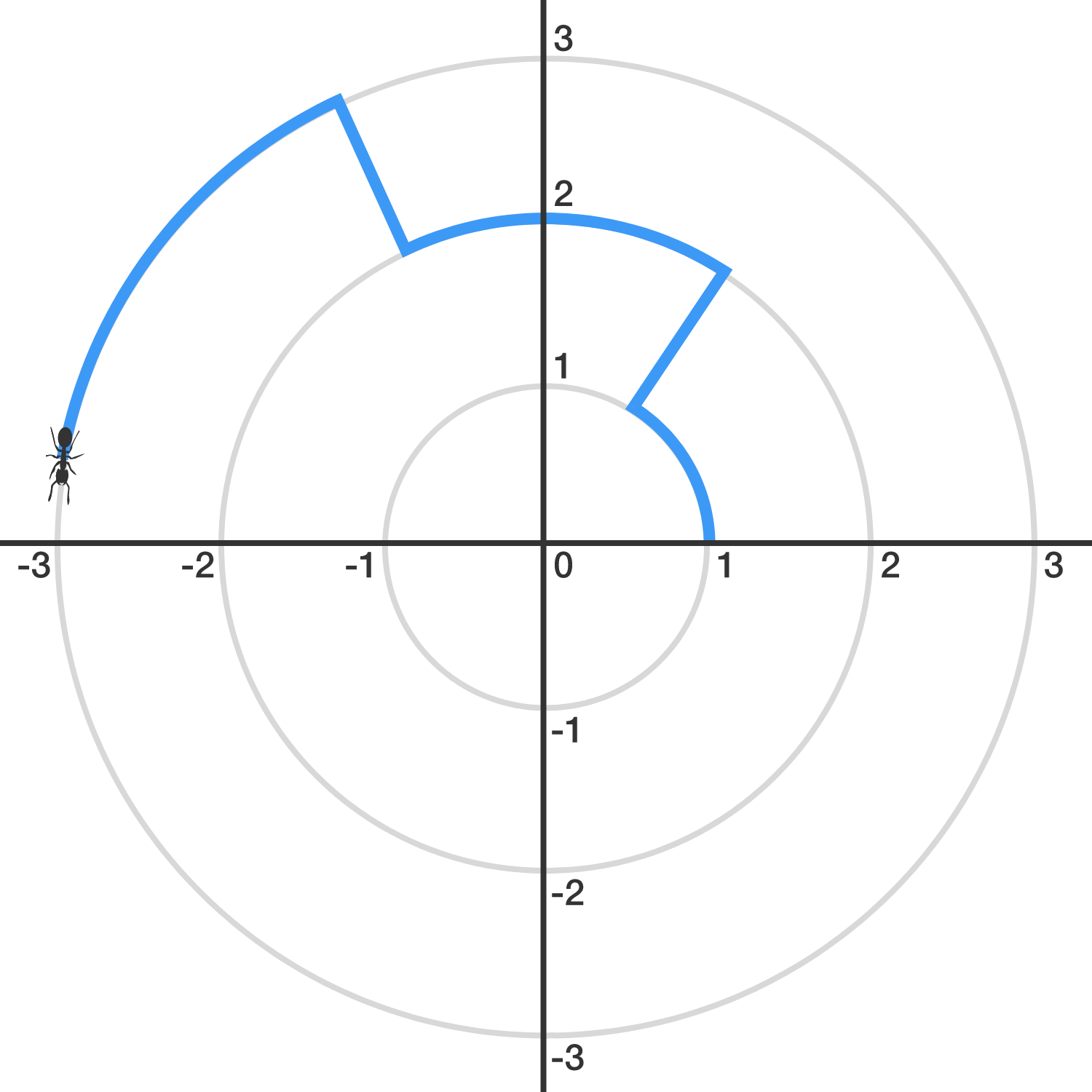# Travelling AntAn ant finds itself trapped in the $xy$-plane, and its initial position is $(1,0).$

Let $S_k$ denote the circle with radius $k$ centered around the origin. Starting from $(1,0)$, the ant walks 1 unit counter-clockwise on $S_{1}.$ Then, it walks directly (radially outward) to $S_2,$ on which it will walk 2 units counter-clockwise. Then, it will walk directly to $S_3$ and walk 3 units counter-clockwise, and so, with the ant walking $k$ units on $S_k.$ (See the image above.)

When the ant crosses the positive $x$-axis for the first time since it left $(1,0)$, it is on $S_{n}$. What is $n$?

×